Type Here to Get Search Results !

# PHYSICS FORM THREE TOPIC 4: OPTICAL INSTRUMENTS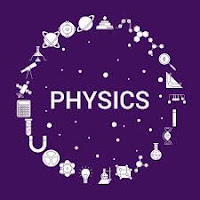OPTICAL INSTRUMENTS
The Structure of the Simple Microscope
Describe the structure of the simple microscope
A magnifying glass, an ordinary double convex lens with a short focal length, is a simple microscope. The reading lens and hand lens are instruments of this type. When an object is placed nearer such a lens than its principal focus, i.e., within its focal length, an image is produced that is erect and larger than the original object. The image is also virtual; i.e., it cannot be projected on a screen as can a real image.The Mode of Action of a Simple Microscope
Describe the mode of action of a simple microscope
The image formed by magnifying glass or simple microscope is virtual and erect object place between principal focus (f) and convex lens.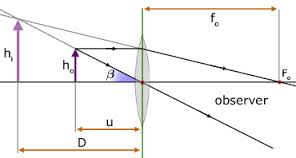• The normal district vision
• The position of the lens is usually adjusted so that V is about 25cm, which is the shortest distance of distinct vision.
Using the equation of lens (Lens formula).
I/U + I/V = I/F
Adopting the 'real is positive' sign convention we obtain:
V = (-Ve) since the image is virtual.
I/U – I/V = I/F
V= 25 –(Normal district vision)
I/U – I/25 =I/F
I/U = I/F + I/25
(I/U)=-1 (25 + F )
25F
U = 25F/F+25
The above formula shows the means of obtaining the distance of object, U.
Magnication (M) of simple microscope
Magnification is the ratio of the image distance to the object distance.
M = Image distance, V
Object distance, U
Hence
M = v/u …………………..(i)
From V = 25cm (distance of district vision)
From U = 25f/(f+25) ……………………… (ii)
Insert eqn (ii) into (i)
M = V/ (25f/(f+25)
M = 25/(25f/f+25)
M = 25/f + 1
Example 1
A simple microscope with lens of focal length 5cm is used to read division of a scale 0.5mm in size. How large will the division be seen through the simple microscope?
Data given
• Focal length, f = 5cm
• Required to find magnification, M
Soln:
From
M = (25/f + 1)
= (25/5+1)
=(5+1)
= 6
The magnification of lens = 6
Let the size of the object be ho and that of the image be hi. Then:
M = h1/H ……………(i)
H1 = 6h
The Height , h = (0.5mm)
H1 = 6 (0.5mm)
HI = 3mm
Hence, each division will appear to have a size of 3.0mm viewed through the simple microscope.
A Simple Microscope
Construct a simple microscope
Parts of simple microscope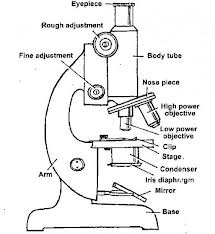The Structure of a Compound Microscope
Describe the structure of a compound microscope
A compound microscope is an optical instrument used to produce much greater magnification than that produced by simple microscope. The main features of a compound microscope includes two short-focus convex lenses, the objective lens, and the eyepiece.
DemonstrationThe Mode of Action of a Compound Microscope
Describe the mode of action of a compound microscope
The most commonly used microscope for general purposes is the standard compound microscope. It magnifies the size of the object by a complex system of lens arrangement.
It has a series of two lenses; (i) the objective lens close to the object to be observed and (ii) the ocular lens or eyepiece, through which the image is viewed by eye. Light from a light source (mirror or electric lamp) passes through a thin transparent object.
The objective lens produces a magnified ‘real image’ (first image of the object). This image is again magnified by the ocular lens (eyepiece) to obtain a magnified ‘virtual image’ (final image), which can be seen by eye through the eyepiece. As light passes directly from the source to the eye through the two lenses, the field of vision is brightly illuminated. That is why it is a bright-field microscope.
The Magnification of a Compound Microscope
Determine the magnification of a compound microscope
The object lens forms a real and inverted image IIof the object O ( the image is slightly magnified). The eyepiece lens acts as a magnifying glass for the first image II and produces a magnifical virtual image.
The object is placed just beyond the principal (fo) of the objective lens so that that the real image I, is formed inside the principal focus (F) of the eye piece. The eyepiece treats the real image I, as an object and then forms its magnified virtual image I2.
Magnification of a compound microscope: This isthe ratio of the image distance produced by a compound microscope to the object distance. The magnification produced by objective lens is v/u.
Where
V is the image distance
U is the object distance
The magnification given by the eyepiece is given by;
Me = 25/fe + 1
If the final image is formed at the least distance of distinct vision (V = 25cm).
Mc = Mome
Combine eqn (i) and (ii)
Then
Mc = (v/u) (25/fe+1)
The above formula shows that the final virtual image is formed at the least distance of distinct vision.
Uses of a Compound Microscope
Mention uses of a compound microscope
The uses of a compound microscope includes the following:
• Used to magnify microorganism such as bacteria which cannot be seen by naked eyes.
• Used in hospitals widely to detect microorganisms in specimens provided by patients. A specimen is a small amount that is taken for testing. Blood is an example of specimens. In hospitals microscopes can detect parasites such as plasmodium ssp (a causative agent for malaria) in blood specimen.
Example 2
A certain microscope consists of two converging lenses of focal length 10cm and 4cm for the objective and eyepiece, respectively. The two lenses are separated by a distance of 30cm. The instrument is focused so that the final image is at infinity. Calculate the position of the object and the magnification of the objective lens.
For the objective lens
I/U + I/V = I/Fo
Where
Fo = 10cm
The objective lens forms a real image of the object at the principal focus of the eyepiece.
Thus
V = (30 – 4)
= 26cm
Thus I/U + I/V = I/10
I/U + 1/26 = 1/10
1/U = (1/10 – I/26)
(I/U) -1 = (4/65)
(1/U) -1 = (4/65)-1
U = (65/4)
The distance of object, U= 16.25cm
The magnification given by the objective lense is given by:
Whereas:
V = 26cm
U= 16.25cm
Mo = (26cm/16.25cm)
The magnificent given by objective lens, Mo = 1.6.
The Structure of an Astronomical Telescope
Describe the structure of an astronomical telescope
An Astronomical Telescope is used for observing heavenly bodies like stars and planets (generally bodies which are very far away from normal vision of human eyes ). Like compound microscope, it consists of two convex lenses, objective lens and the eyepiece.
The focal length Fb of the objective lens is longer than the focal length Fe of the eye piece lens.Rays of light from a distant object are nearly parallel when they strike the objective lens of the Telescope.The objective lens forms a real image, inverted and diminished image IQ of a distant object is in the focal plane.The eye piece forms the final magnified image at infinity
When the telescope is adjusted in such a way that the final image is at infinity it is said to be in normal adjustment.In this case the distance between objective lens and eyepiece is (Fb + Fe) This is the maximum separation between the objective lens and the eyepiece lens.
The Mode of Action of an Astronomical Telescope
Describe the mode of action of an astronomical telescope
The main reason for a distant object to be smaller is that the two objects subtend different angles at the eye. In other words, we can say that different angles substended by the eye causes a distant object to appear smaller.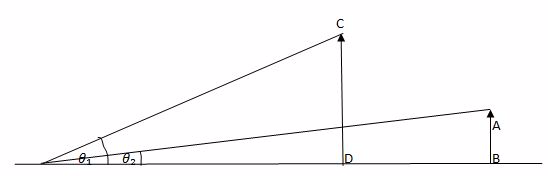The object AB and CD are of the Same height.The object CD is closer to the eye than AB.
The object CD appears to be taller than AB because angle B that CD subtends at the eye is greater than the angle x subtended by AB at the eye. Images there can be made to appear large by bringing them closer to the eye.
In a telescope the final image is magnified because it subtends a much greater angle at the eye than does a distant object observed without a telescope. B is the angle subtended by the final image at the eye and X is the angle subtended by a distant object.
The Magnification of an Astronomical Telescope
Determine the magnification of an astronomical telescope
The magnification of a telescope is defined as the ratio of the angel B (in radians) subtended by the final image at the eye to the angle X subtended by a distant object at the eye.
Thus, for telescope the magnification is given by:
M = B/x ………………………………….i
From figure B= IQ/ID ……………………..ii
X = IQ/IA ………………………………………..iii
But Insert eqn (ii) and (iii) into eqn (i)
M = (12/ID)
(IQ/IA)
M = (IA/ID)
But IA = fo and IF =fe
M = fo/fe……………………………….(x)
Where
Example 3
fois the focal length of two thin converging lenses of focal lengths 25cm and 4cm respectively. It is focused on the moon which subtends an angle of 0.6° at the objective lens. The final image is formed at the observers least distance of distinct vision (25cm in front of the eyepiece). Find the diameter of this image.
In the previous figure:
X = h/fo
Where fo is the focal length of the objective lens
X = h/25
Where X is the angle in radians subtended at the objective lens by the moon.
H = 25x
H = 25 (6/10 x 11/180)
H = 25 (6/10 x 22/7 x 1/80)
H = 0.2619m
The height of the image, h = 0.2619m
The distance of this image from the eyepiece is obtained from the relation:
• I/U + I/V = I/fe = 4cm
• V= -25cmV = -25cm
• I/U – I/25 = ¼
• I/U = (1/4 + 1/25)
• (I/U)-1 = (25 +4) -1/100
• 100U= (100/29)
The magnification, m of the lens:
• M = V/u
• M = (25CM/100/29)
• M = 29/4
Let the height of the final image of the moon be h:
• M = Hi/h
• hI = mh
• HI = (29/4) (0.2619)
• HI= 1.90cm
The Height of image Hi = 1.9cm
Hence
The diameter of the final image of the moon will be 1.90cm
Observation of the universe today are best made from the Hubble Telescope. Outside the Earth’s atmosphere, this telescope suffer from less interference.
Uses of an Astronomical Telescope
Mention uses of an astronomical telescope
Astronomers use telescopes because they're much better than our eyes. Here are a few reasons:
1. Telescopes see lots of colours - telescopes can collect light that our eyes are unable to: radio, microwave, infrared, ultraviolet, x-rays and gamma rays.
2. Telescopes collect lots of light - our pupils are only a few millimeters across, so we can only collect photons over a tiny area whereas telescopes can collect photons of huge areas (e.g. a football fields worth for radio telescopes).
3. Telescopes see fine details because of the wave nature of light and the nerves in our eyes, we can only see details about the same angular size as Jupiter's width. Telescopes can allow us to resolve fine details - like Jupiter's Great Red Spot.
4. Telescopes can record observations with cameras - You can see things with your eye and draw them, but telescopes can share observations with the world! This is especially important for convincing skeptics that what you saw was real!
A Simple Astronomical Telescope
Construct a simple astronomical telescope
A simple telescope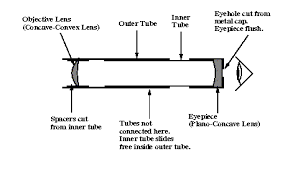The Structure of the Projection Lantern
Describe the structure of the projection lantern
The projection lantern forms images of slides or camera film onto a distant screen. The film or slide to be projected is inverted and highly illuminated.
The Mode of Action of a Projection Lantern
Describe the mode of action of a projection lantern
Optical arrangement of projection lantern.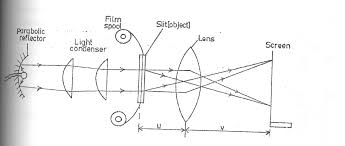• The slice or film to be projected is inverted and highly illuminated.
• The concave mirror helps to concentrate the light which would otherwise be partly wasted.
• The lamp is placed at the principal focus of the concave mirror.
• The heat filter reduces the heat at falling on the slide or film so as to avoid it overheating.
• Since the image of the projection lantern is Highly magnified, it would not be very bright if there was not enough illumination.
• The condenser directs a maximum amount of light from the source of the slide and produce uniform illumination the screen. (The condenser is a double in order to reduce chromatic aberration).
• The projection lens forms the image of the slide on the screen.
• The light source is usually located at a distance of 2f from a condenser and invited so that the image on the screen is upright (erect).
• The focal length of the projection lens is ABOUT TWICE THE FOCAL length of the condenser since the screen is usually far from the lens.
The Magnification of a Projection Lantern
Determine the magnification of a projection lantern
Example 4
A lantern projector using a slide of (2cm x 2cm) projects a picture (1cm x 1cm) onto a screen 12m from the projection lens. How far from the lens must the slide be? Find the approximate focal length of the projection lens.
Solution
The magnification m is given by;
M = V/U ………………………………………i
Where
• His the size of image
• H is the size of object
• U object distance
• V image distance
Thus
M = Hi …………………. ii
Then eqn (i) = eqn ii
• v/u =hi/h
• (1200/u)-1 = (100/2) -1
• (u/1200) = (2/100)
• U = (2/100) (1200)
• U = 24cm
The object distance, U = 24cm
Uses of a Projection Lantern
Mention uses of a projection lantern
Projection lantern are used in various areas. These include:
• Projection of films, slides and transparencies,
• projection of opaque objects, i.e. episcopic projection,
• used in searchlights and headlights,
• used in projection apparatus in industry for gauge and screw thread testing,
• used in physical experiments such as projection of a spectrum,
• used in polarisation experiments and interference experiments.
A Simple Projection Lantern
Construct a simple projection lantern
Projection Lantern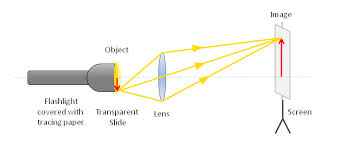The Structure of the Lens Camera
Describe the structure of the lens camera
Lens camera is an instrument which produces an image of object on the screen using light. The basic physical principle of all camera is the same in spite of the variation in the design of cameras.
The optical system of the camera are very similar to that of the lantern projector but with the direction of light reversed.The converging lens forms a real image of the object to be photographed.(This image is diminished (smaller than the object and inverted)
The lens can be moved back and forward with the help of focusing any so that objects at different distances can be brought to the focus.A forced image is locate on the film or plate when the shuttled is open for a suitable amount of time as determined by the shutter speed.
Light enters the camera Box and makes a picture of the object on the film “( The film is sensitive to light)
The camera is equipped with a diagram or light entering the camera.It ensures that is incident centrally on the lens so that the distortion of the image formed is reduced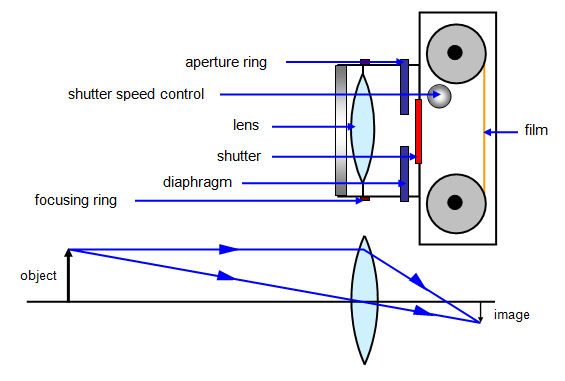The Mode of Action of the Lens Camera
Describe the mode of action of the lens camera
The aperture stop, which is the limiting diameter of the aperture thought which light enters the camera (given as fraction of focal length F of lens) is also called F Number.
This F Number; is the fraction of focal length of the lens given as focal length divide by lens diameter.
F number = Focal length, F/Lens diameter, d
FN = F/d
Where d = is lens diameter.
• The Number Indicates the Number of times the focal length F of times the focal length F of the lens diameter ( or stop)
• The smaller the F - Number for a given focal length the larger the lens diameter
• The lens with a larger diameter has a greater light- gathering power or speed
• This for such a lens the shutter allows light in the camera for a short interval of time.
The Magnification of the Lens Camera
Determine the magnification of the lens camera
Magnification of a lens camerais obtained as the ratio of the Image distance and the object distance.
But from the lens formula:
Thus M = v/U
I/U + I/V = I/F
I/V = I/F - I/U
(I/V) –I = ( U - F / FU)-I
V = FU/ ( U - F)
Example 5
A lens camera is to be used to take a picture of a man 2m tall if the lens of the camera Has a focal length of 10cm, calculate the minimum size of the film frame required, given that the man is 20.1m from the camera.
Solution:
Magnification is given by:
M = f/ (u-f)
Where
F= 10cm U = 201/m / 2010cm
M = ( 10/2010 – 10)
M = 1/20 ....................................i
Let the size of the frame be h when the height of man is 2m.
Then
M = 1/200
But h1/h = 1/200
h1 = (1/200) 2
h1 = (1/200)2
h1 (2/200)
h1 = (1/100) m
h1 = 1cm or 10mm
The film frame should be at least 10mm square.
Simple Lens Camera
Construct a simple lens camera
A simple lens camera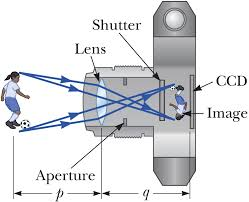The Structure of the Human Eye
Describe the structure of the human eye
The eyeball approximately spherical in shape.The wall of this sphere consist of two layers, the outer layer or sclera and the inner layer or choroid.The front portion of the SCLERA FORMS A TRANSPARENT CURVED section called the camera.The choroid layer is balance in order to prevent internal reflection and also to protect the light sensitive parts of the eye.
The aqueous and vitreous hum our are jelly – like substance that fills the spaces within the eyeball.The aqueous humour is the salt solution of refractive index n, 1.38.Vitrous hurmour is a watery , Jelly substance of refractive index 1.34.Behind the cornea there is a colored diagram called the iris.
The iris has the central hole called the pupil. The iris contains muscles which control the size of the pupil. The size of the pupil decreased in the bright light and increased in the dim light.
Behind the pupil and there is a crystalline lens held in position by suspensory ligaments that are attached to the choroid layer.Near the suspensory ligaments are the ciliary muscles.The function of the suspensor ligaments there are the cilliary muscles.
The function of cillary muscles is to control the thickness of the lens. The lens become thick when the ciliary muscles contract and thin when the ciliary muscles are relaxed.
At the back of the eye there is a retina (This is the part of the eye which is sensitive to light).Image formed is inverted formed on the Retina ( This is the part of the eye which is sensitive to light.)
Image formed is inverted formed on the retina by successive refraction of light at the corner, the aqueous hurmour the crystalline lens and the Vitreous hurmour.Electrical signals are then transmitted to the Brain through the topic nerve. Finally, the brain interprets these signals.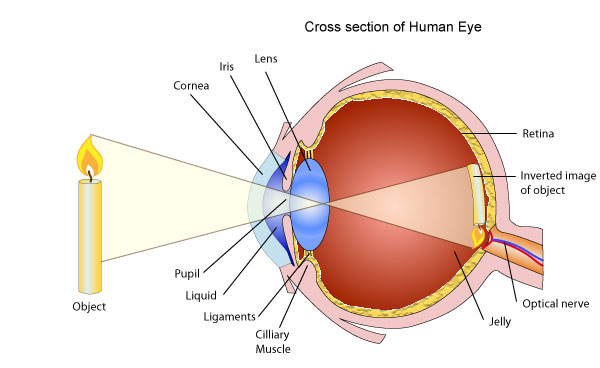Accommodation Power of the Human Eye
Explain accommodation power of the human eye
Accommodation is the process whereby the eye alters its focal length in order to form images of objects at different distances.
(Thickening or Thinning of the lens causes a change in its focal length).
The thickening or thinning of the crystalline lens is made possible by the action of the ciliary muscles.To view neare object t, ciliuary muscles contract, this makes the lens thicker.
In the relaxed state of ciliary muscles, the crystalline lens become thinner and enables the eye to see (view) distant objects. The farthest point which can be seen clearly is called the far point of the eye and the nearest point is called the near point of the eye.
The corresponding distance from these points to the eye are referred to as the maximum and least distance of district vision respectively.A normal eye (i.e. without defects of vision) has a far point at infinity and near point at a distance of 25cm from the eye.Structure of lens “ view distant object”
The Defects of the Human Eye
Identify the defects of the human eye
Myopia or near-sightedness
• This defect causes person to see near object clearly while distant objects are not seen clearly.
• The strength of the cornea and the eye lens combination is too great even when muscles of the eye are completely relaxed.
• The focal length of the cornea and the eye – lens combination is always less than the distance to the retina.
• Images of distant object are formed in front of the retina even when eye is totally relaxed. However, an object that is closer can be brought into focus.
• In this situation the focal length of the cornea and the eye lens is so short that objects closer than the conventional (near point of 25cm) can be brought into focus. That’s why this condition is called Short sightedness (near sightedness).
• Since the problem is that the strength of the eye – lens and the cornea combination is too great, the solution is to provide eye glasses (or contract lenses) with negative lens.
• The negative lens weakens the strength of the cornea and eye – lens just enough so that the resulting focal length when the eye muscles are relaxed matches the distance back to the retina so that distant images are now in focused.
• The eye glass lenses are negative lenses that means they are thinner in the middle than at the edges.
• It is easy to identify this kind of eye glass lenses since acting by themselves they do not form a real image of an object at any distance.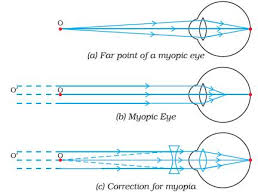Hyperopia or far-sightedness
• This defect causes a person to see distant objects only and short-distance objects are not seen clearly.
• In the person with this condition, the strength of the cornea and the eye-lens combination is too weak when the eye muscles are totally relaxed. So the image of a distant object is formed behind the retina.
• The solution in the opposite of myopia. Victims should wear positive eye lenses which strengthen the corner and the eye lens just enough so that the resulting focal length when the eye is relaxed matches the distance to the back of the retina.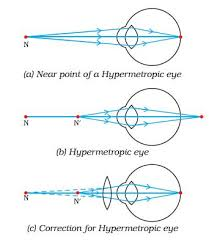Astigmatism
• This occurs when the focal length for the cornea and the eye's lens for an object oriented in some direction is not the same as for another located in a perpendicular direction.
• The eye can not bring the vertical and horizontal lines in a ‘+’ symbol in sharp focus at the same time. (The axis of differing focal length need not be exactly horizontal and vertical).
• The problem is that the cornea of the eye lens is not symmetrical. The solution is to use eye glasses whose lenses are not symmetrical in a complementary way.
• The cylindrical lens may be combined with an additional positive or negative lenses.
Decreased accommodation
• This condition typically occurs in middle-aged people.
• The eye muscles gradually weaken with age, so that the range or accommodation is decreased.
• People with this condition cannot bring both near objects and far objects into focus.
• The weakening of the eye muscles often causes the focal length of the eye lens to increase as well so that many people of middle age tend to become far sighted.
• Since the problem is adequate accommodation, no single lens can correct it and people with this problem usual needs bifocals.
• Bifocals are glasses with two different lens strengths, one for near and one for distant objects.
• The usual arrangement is that the bottom half of the lens is the near strength and the top half is the far strength.
The Correction of the Defects of Human Eye
Describe the correction of the defects of human eye
Myopia is common name for impaired vision in which a person sees near objects clearly while distant objects appear blurred. In such a defective eye, the image of a distantobject is formed in front of the retina and not at the retina itself. Consequently, a nearsighted person cannot focus clearly on an object farther away thanthe far point for the defective eye.
This defect arises because the power of the eye is too great due to the decrease in focal length of the crystalline lens. This may arise due to either
1. excessive curvature of the cornea, or
2. elongation of the eyeball.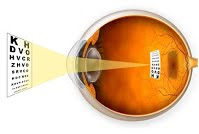Correction:Thisdefectcan becorrectedby using aconcave (diverging) lens. A concave lens of appropriate power or focal length is able to bring the image of the object back on the retina itself.
Farsightedness, also called hypermetropia, common name for a defect in vision in which a person sees near objects with blurred vision, while distant objects appear in sharp focus. In this case, the image is formed behind the retina.
This defect arises because either
1. the focal length of the eyelens is too great, or
2. the eyeball becomes too short, so that light rays from the nearby object, say at point N, cannot be brought to focus on the retina to give a distinct image.
Correction:This defect can be corrected by using aconvex(converging) lensof appropriate focal length. When the object is at N’, the eye exerts its maximum power of accommodation. Eyeglasses with converginglenses supply the additional focussing power required for forming the image on the retina.
The Human Eye and the Lens Camera
Compare the human eye and the lens camera
The camera
1. The eye and the camera has a have a convex lens which form a real and inverted image of an object.
2. The eye and the camera are blackened inside to prevent internal reflection. Rays of light which are not received on the retina or camera film are absorbed by the choroid layer of the eye or the black surface inside the camera.
3. The eye can regulate the amount of light that passes through the crystalline lens by using pupil while in a camera the diaphragm regulates light.
4. In the eye the image is formed in the retina while in the camera the image is formed on the photographic plate.
5. The eye can change the focal length of its lens by the contraction and relaxation of the ciliary muscles. In this way the eye can focus objects at different distance. In a camera objects at different distance are focused on by moving the lens forwards and backwards.

Tags